A spreadsheet originally existed in the physical world as a piece of paper that you could actually write on. These paper spreadsheets were used mainly by Accountants to help organize data in a logical and easy to view manner. However, the spreadsheets of the Information Age reside on your computer, though they still have the basic form of the old spreadsheets.

This lesson will teach you about the different elements that make up a spreadsheet, including some vocabulary so that you can understand the following lessons of Tizag's Excel Tutorial. We have included several diagrams of actual Excel spreadsheets, which we encourage you to compare to the Excel program you are running at home or in the office.

The spreadsheet (or worksheet) contains or will contain all the data that you enter. When you start up Excel, you should be greeted with a blank spreadsheet like the one we have below.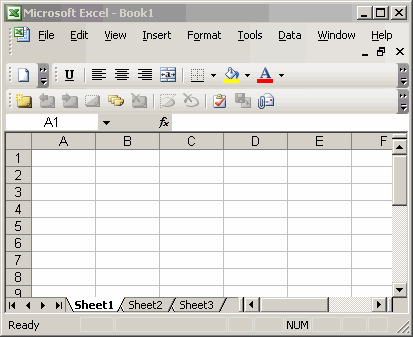Nearly every part of the spreadsheet has its own term and it is quite useful to know your spreadsheet vocabulary to make using Excel that much easier. There are three basic parts of an Excel Spreadsheet:

1. Column - The vertical segments that you see on the spreadsheet are called columns.
2. Row - The horizontal segments are referred to as rows.
3. Cell - Each box that is created from a row and column intersecting is referred to as a cell.

There are all very important terms, so we will now be going into greater depth on exactly what they are.

Excel Columns

Each vertical segment of the spreadsheet is referred to as a column. Here is the "A" column.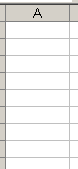Excel uses letters to represent columns and numbers to represent rows. This may be a little confusing at first, but with practice and a little effort this will become second nature to you.

Excel Rows

The horizontal segments, those that run left-to-right, are referred to as rows. Here is row "1".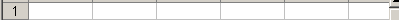Once again, Excel uses numbers to label rows.

Excel Cells

Now that you understand columns and rows you can grasp the labeling system for cells. When a column and a row intersect they form a cell. The very first cell, where column A and row 1 intersect is referred to as A1. It seems that the column label always comes first and the row second. Try to remember that!

Here's a picture of Cell A1: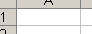If you are ever having trouble trying to find a cell, we recommend the following technique (we use cell G13 as an example):

1. Find the column on the column bar by looking for the letter of the cell (in this case that would be G).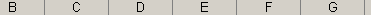2. Find the row on the row bar by looking for the number of the cell (in this case that would be 13).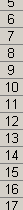3. After you have found both the column and the row trace an invisible line from each to the cell where they intersect and click there if you wish to enter data.

Found Something Wrong in this Lesson?

Report a Bug or Comment on This Lesson - Your input is what keeps Tizag improving with time!

 Web Reference HTML Reference CSS Reference CSS Examples PHP Examples Help Tizag Grow Link to Tizag Tizag.com Store
 Advertise Here More Tutorials! Microsoft Office Tutorials Artist Tutorials